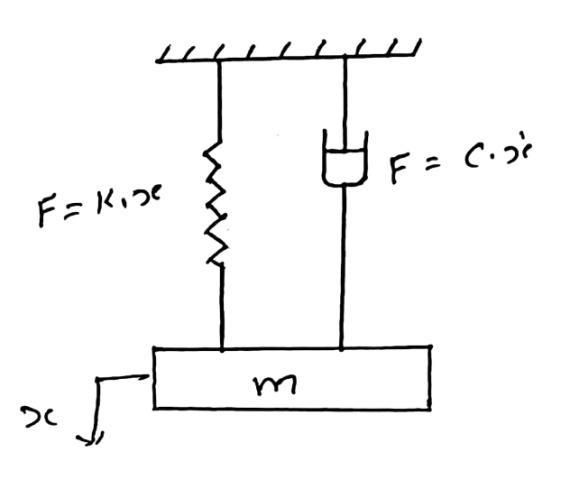## Damped free Vibration-Numerical 1

#### A mass of 85 kg is supported on a spring which deflects 18 mm under the weight of the mass. The vibrations of the mass are constrained to be linear and vertical. A dashpot is provided which reduces the amplitude to one-quarter of its initial value in two complete oscillations. Calculate magnitude of the damping force at unit velocity and periodic time of damped vibration.

Solution:Fig-1

Given data:

mass $\ m = 85$ kg
Static deflection, $\ x = 18\ \text{mm} = 0.018\ \text{m}$

Let,
$x_0 =$ initial amplitude
$x_2=$ final amplitude after two complete cycle $= {1\over4}$

Taking ratio,

${x_0 \over x_2}={x_0 \over {1 \over 4} x_0}=4$

#### Damping force:

$\text F = c.\mathring x= c$

Now,

\begin{aligned} \xi= {c \over c_c} \quad \text {OR} \quad &\xi= {c \over 2m\omega_0} \quad (\text {as} \quad c_c=2m\omega_n)\\ \therefore \ &c=(2m\omega_n)\xi \ \dots\dots\dots\dots \ (1) \end{aligned}

#### 1. The Logarithmic decrement:

\begin{aligned} \delta &= {1 \over n} log_e\bigg ({x_0 \over x_n}\bigg)\\ &= {1 \over 2} log_e\bigg ({x_0 \over x_2}\bigg)\\ &= {1 \over 2} log_e(4) \end{aligned}

#### 2. Damping factor:

\begin{aligned} \xi &= {\delta \over \sqrt{4 \pi^2 + {\delta}^2} } \\ &={\delta \over \sqrt{4 \pi^2 + {\delta}^2} }\\ &= 0.1091 \end{aligned}

#### 3. Frequency of undamped free vibration:

The natural circular frequency of vibration is,

\begin{aligned} \omega_n &= {\sqrt{k \over \ m}} \\ &= {\sqrt{m \ g \over \ x \ m}}\\ &= {\sqrt{g \over x}} \\ &= {\sqrt{9.81 \over 0.018}}\\ &= 23.34 \ \text {rad/s} \end{aligned}

From equation (1); we get,
Damping force at unit velocity;

\begin{aligned} \text F = c &= (2m\omega_n)\xi \\ &= (2 \ \text x \ 85\ \text x \ 23.34)\ \text x \ (0.1091) \\ &= 91.67 \ \text N \end{aligned}

The time period of damped vibration is,

\begin{aligned} t_p &= {2\pi \over \omega_d} \\ &= {2\pi \over \omega_n \ \sqrt {1 -\xi^2}} \\ &= {2\pi \over (23.34) \ \sqrt {1 -(0.1091)^2}} \\ &=0.2708 \ \text {sec} \end{aligned}